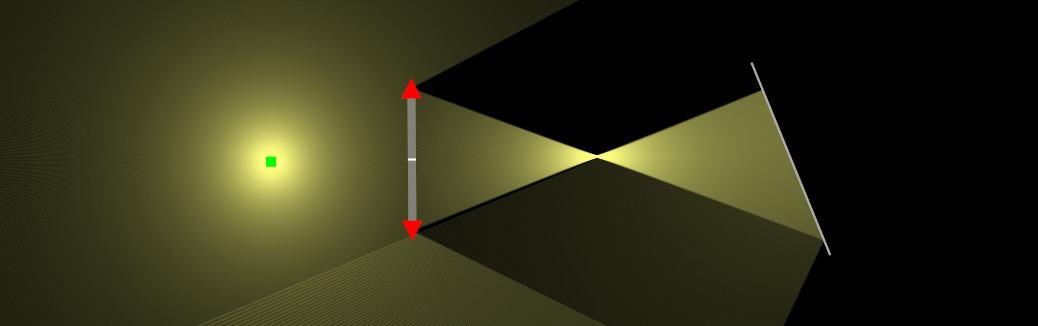﻿ Ray Optics Simulation - PhyDemo

# Ray Optics Simulation

Create & simulate 2D geometric optical scenes interactively.
Totally free and web-based. Source code is available on GitHub.

## ToolsRay

A single ray of light defined by two points.Beam

A parallel beam of rays emerges from a line segment, with density controlled by the "Ray density" slider.Point Source

Rays emerge from a single point, with number controlled by the "Ray density" slider.Mirror

Simulate the reflection of light on a mirror.Mirror (Curved)

A mirror whose shape is curved. Can be circular, parabolic, or defined by a custom equation y = f(x).Ideal curved mirror

The idealized "curved" mirror which obeys exactly the mirror equation (1/p + 1/q = 1/f). The focal length (in pixels) can be set directly.Beam Splitter

A mirror that transmits a percentage of incoming light.Glass

Simulate the refraction and reflection of light on a surface.Glass (Other shapes)

Glass with any shapes constructed from line segments and circular arcs, or shapes defined by a custom inequality f(x) < y < g(x).Glass (Ideal lens)

An ideal lens that obeys exactly the thin lens equation (1/p + 1/q = 1/f). The focal length (in pixels) can be set directly.Blocker

A line-segment light blocker that absorbes the incident rays.Ruler

A ruler from a point for zero and another point. The scale is in pixels.Protractor

A protractor defined from the center and another point for the zero direction. The scale is in degrees.Detector

A tool for measuring the rate of energy flow (P), the rate of perpendicular momentum flow (F⊥), and the rate of parallel momentum flow (F∥) through a line segment. The units are arbitrary.

## Views

Rays

Show the rays. When the "Ray density" is high, they appear to be continuous.Extended Rays

Show both the rays and their extension. Orange indicates backward extensions, and gray indicates forward ones.All Images

Show the position of all images. Yellow points indicate real images, orange indicates virtual images, and gray (none in this picture) indicates virtual objects. Note that some images cannot be detected if "Ray density" is not high enough.Seen by observer

Simulate the rays and images seen from some position. The blue circle is the observer. Any rays crossing it are considered to be "observed". The observer does not know where the rays actually begin, but may think they begin at some point(s) if they intersect there. The rays are shown in blue, and the point(s) in yellow (real) or orange (virtual).Simulate Colors

Simulate colors (wavelengths) of light sources, mixture of colors, color filtering, and chromatic dispersion of glasses.